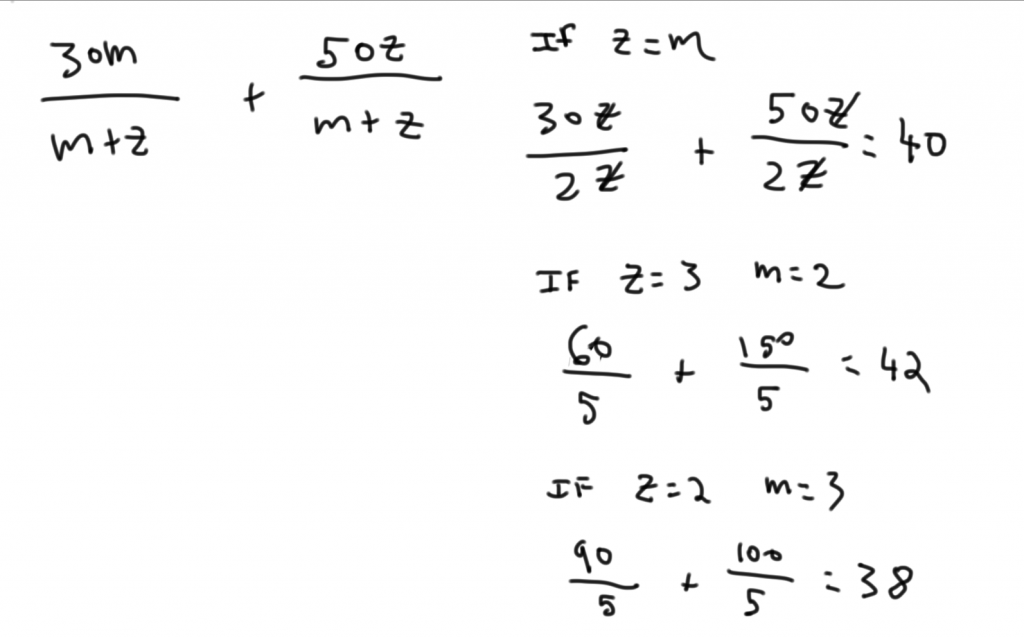Private GMAT Tutoring in NYC & Online

# GMAT Question of the Day - Data Sufficiency - Inequalities

If m and z are positive numbers is 30m/(m+z) + 50z/(m+z) greater than 39?

(1) z/m > 1

(2) m/(m+z) < 1/2

## GMAT Question of the Day Solution:

Look at this as a weighted average question. If m = z then the answer will end up in the middle of 30 and 50. In this case 40. So the real question here is: z > m?  If this is confusing then I would suggest trying some numbers to make this concept very clear. Make  z=m, z > m, and finally m > z. Hopefully that will shed some light on how this question works (I worked this out at the bottom).

(1) Simplify the algebra to z > m. If z is greater than m then the value of the expression must be closer to 50 than 30. So the expression must be greater than 39. Sufficient.

(2) If the first part of the expression has less than half of the weight than the total expression must be closer to 50 then 30. So the expression must be greater than 39. Sufficient.# CONTACT

Atlantic GMAT

405 East 51st St.

NY, NY 10022

(347) 669-3545

info@AtlanticGMAT.com